# 一类耦合Burgers方程组的数值计算Numerical Solution to Coupled Burgers’ Equations

DOI: 10.12677/AAM.2019.812225, PDF, HTML, XML, 下载: 193  浏览: 300  科研立项经费支持

Abstract: This paper solves a class of coupled Burgers’ equations based on finite volume scheme in a uniform grid. A new scheme for discretizing convective term is constructed by Hermite interpolation method with satisfying TVD (Total Variational Diminishing) criterion, and the time discretization is fulfilled by using the third-order Runge-Kutta scheme. It is verified that this scheme has gained a good computational performance by several classical numerical examples.

1. 引言

$\frac{\partial u}{\partial t}-\delta \frac{{\partial }^{2}u}{\partial {x}^{2}}+\eta u\frac{\partial u}{\partial x}+\alpha \frac{\partial \left(uv\right)}{\partial x}=0,\text{\hspace{0.17em}}x\in \Omega =\left[a,b\right],\text{\hspace{0.17em}}t\in \left[0,T\right]$

$\frac{\partial v}{\partial t}-\mu \frac{{\partial }^{2}v}{\partial {x}^{2}}+\xi v\frac{\partial v}{\partial x}+\beta \frac{\partial \left(uv\right)}{\partial x}=0,\text{\hspace{0.17em}}x\in \Omega =\left[a,b\right],\text{\hspace{0.17em}}t\in \left[0,T\right]$

$u\left(x,0\right)={g}_{1}\left(x\right),\text{\hspace{0.17em}}x\in \Omega =\left[a,b\right]$

$v\left(x,0\right)={g}_{2}\left(x\right),\text{\hspace{0.17em}}x\in \Omega =\left[a,b\right]$

$u\left(a,t\right)={f}_{1}\left(t\right),\text{\hspace{0.17em}}u\left(b,t\right)={f}_{2}\left(t\right),\text{\hspace{0.17em}}t\in \left[0,T\right]$

$v\left(a,t\right)={f}_{3}\left(t\right),\text{\hspace{0.17em}}v\left(b,t\right)={f}_{4}\left(t\right),\text{\hspace{0.17em}}t\in \left[0,T\right]$

Esipov  推导了一维粘性Burgers方程，研究了多分散沉降模型。该耦合方程是重力作用下两种颗粒在流体悬浮液或胶体中的沉降或单位体积浓度(scaled volume concentrations)演化的简单模型。Burgers  和Cole  发现，该方程组描述了各种现象，如湍流的数学模型和在粘性流体中流过激波的近似理论。Soliman  采用了改进的扩展的Tanh函数法得出一维耦合Burgers分解法的精确解，但在一般情况下，耦合burgers方程的解析解是不可用的。很多研究人员对一维耦合Burgers方程的数值解进行了求解。 Esipov给出了一些数值模拟，并将结果与实验数据进行了比较。Abdou和Soliman  用变分迭代法求解一维Burgers方程和耦合Burgers方程。Deghan  等人利用Adomian-Pade技术，得到了粘性Burgers耦合方程的数值结果。Mittal和Arora  提出了一种基于Crank-Nicolson和三次B样条函数格式的耦合Burgers方程数值求解方法。Khater  等提出了非线性偏微分方程近似解的Chebyshev谱配置法，利用Chebyshev谱配点法，将问题简化为一个ODES系统，然后用四阶Runge-Kutta方法求解。Rashid和Ismail  等人利用空间上的Fourier伪谱法和经典的四阶Runge-Kutta方法，给出了具有一组初值、周期边界条件的耦合Burgers方程的近似解。Mittal  等人用多项式微分求积法(PDQM)分析了一个耦合Burgers方程。PDQM将耦合Burgers方程转化为非线性方程组，然后用四阶Runge-Kutta方法求解。Mohanty  等人提出了一种求解耦合Burgers方程的四阶隐式紧算子方法，没有使用任何变换或线性化技术来处理非线性项。Arminjon和Beuchamp  提出了二维耦合Burgers方程的有限元方法，并得出了与其他方法，即直线法和Runge-Kutta型方法相比，该方法是有效的。魏  等人提出了空间离散的分布式逼近泛函格式和求解一维和二维Burgers方程的Taylor级数展开方法。

2. 数值格式的构建

2.1. Burgers方程

$\frac{\partial u}{\partial t}+u\frac{\partial u}{\partial x}=\nu \frac{{\partial }^{2}u}{\partial {x}^{2}},\text{\hspace{0.17em}}x\in \Omega =\left[a,b\right],\text{\hspace{0.17em}}t\in \left[0,T\right]$ (2.1)

${x}_{\frac{1}{2}}=a<{x}_{\frac{3}{2}}=a+\Delta x<\cdots <{x}_{N+\frac{1}{2}}=b$ (2.2)

${\int }_{{I}_{j}}\frac{\partial u}{\partial t}\text{d}x+{\int }_{{I}_{j}}\frac{\partial f}{\partial x}\text{d}x={\int }_{{I}_{j}}\nu \frac{{\partial }^{2}u}{\partial {x}^{2}}\text{d}x$

$\frac{\partial }{\partial t}{\int }_{{I}_{j}}u\left(x,t\right)\text{d}x+{f\left(x,t\right)|}_{{x}_{j+\frac{1}{2}}}^{{x}_{j+\frac{1}{2}}}={\nu \frac{{\partial }^{2}u}{\partial {x}^{2}}|}_{{x}_{j+\frac{1}{2}}}^{{x}_{j+\frac{1}{2}}}$ (2.3)

$\frac{\partial }{\partial t}{\int }_{{I}_{j}}u\left(x,t\right)\text{d}x+\left[f\left({x}_{j+\frac{1}{2}},t\right)-f\left({x}_{j-\frac{1}{2}},t\right)\right]=\nu \left[{u}^{\prime }\left({x}_{j+\frac{1}{2}},t\right)-{u}^{\prime }\left({x}_{j+\frac{1}{2}},t\right)\right]$ (2.4)

${\stackrel{¯}{u}}_{j}\left(t\right)=\frac{1}{\Delta x}{\int }_{{I}_{j}}u\left(x,t\right)\text{d}x$

$\Delta x\frac{\partial {\stackrel{¯}{u}}_{j}\left(t\right)}{\partial t}+\left[f\left({x}_{j+\frac{1}{2}},t\right)-f\left({x}_{j-\frac{1}{2}},t\right)\right]=\nu \left[{u}^{\prime }\left({x}_{j+\frac{1}{2}},t\right)-{u}^{\prime }\left({x}_{j+\frac{1}{2}},t\right)\right]$

$u\left({x}_{j+\frac{1}{2}},t\right)={u}_{j+\frac{1}{2}}$

$\Delta x\frac{\partial {\stackrel{¯}{u}}_{j}\left(t\right)}{\partial t}+\left({f}_{j+\frac{1}{2}}-{f}_{j-\frac{1}{2}}\right)=v\left({{u}^{\prime }}_{j+\frac{1}{2}}-{{u}^{\prime }}_{j-\frac{1}{2}}\right)$ (2.5)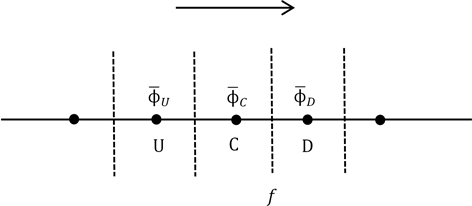Figure 1. Three neighboring mesh points and the mesh face

2.2. 高阶格式

${\varphi }_{f}=f\left({\stackrel{¯}{\varphi }}_{U},{\stackrel{¯}{\varphi }}_{C},{\stackrel{¯}{\varphi }}_{D}\right)$ (2.6)

${\varphi }_{f}$ 可以表达为

${\varphi }_{f}={\stackrel{¯}{\varphi }}_{C}+\left[\frac{1+k}{4}\left({\stackrel{¯}{\varphi }}_{D}-{\stackrel{¯}{\varphi }}_{C}\right)+\frac{1-k}{4}\left({\stackrel{¯}{\varphi }}_{C}-{\stackrel{¯}{\varphi }}_{U}\right)\right]$ (2.7)

$\stackrel{^}{\varphi }=\frac{\stackrel{¯}{\varphi }-{\stackrel{¯}{\varphi }}_{U}}{{\stackrel{¯}{\varphi }}_{D}-{\stackrel{¯}{\varphi }}_{U}}$

${\stackrel{^}{\varphi }}_{f}=f\left({\stackrel{^}{\varphi }}_{U},{\stackrel{^}{\varphi }}_{C},{\stackrel{^}{\varphi }}_{D}\right)$ (2.8)

${\stackrel{^}{\varphi }}_{f}=f\left({\stackrel{^}{\varphi }}_{C}\right)$

${\stackrel{^}{\varphi }}_{f}=\left(1-\frac{k}{2}\right){\varphi }_{C}+\frac{1}{4}\left(1+k\right)$ (2.9)Table 1. The linear convection schemes and the NV formulations

$\left\{\begin{array}{l}{\stackrel{^}{\varphi }}_{C}<{\stackrel{^}{\varphi }}_{f}=f\left({\stackrel{^}{\varphi }}_{C}\right)<1,\text{\hspace{0.17em}}\text{\hspace{0.17em}}\text{\hspace{0.17em}}0<{\stackrel{^}{\varphi }}_{C}<1\\ {\stackrel{^}{\varphi }}_{f}={\stackrel{^}{\varphi }}_{C},\text{\hspace{0.17em}}\text{\hspace{0.17em}}\text{\hspace{0.17em}}\text{\hspace{0.17em}}\text{\hspace{0.17em}}\text{\hspace{0.17em}}\text{\hspace{0.17em}}\text{\hspace{0.17em}}\text{\hspace{0.17em}}\text{\hspace{0.17em}}\text{\hspace{0.17em}}\text{\hspace{0.17em}}\text{\hspace{0.17em}}\text{\hspace{0.17em}}\text{\hspace{0.17em}}\text{\hspace{0.17em}}\text{\hspace{0.17em}}\text{\hspace{0.17em}}\text{\hspace{0.17em}}\text{\hspace{0.17em}}\text{\hspace{0.17em}}{\stackrel{^}{\varphi }}_{C}\le 0\\ {\stackrel{^}{\varphi }}_{f}={\stackrel{^}{\varphi }}_{C},\text{\hspace{0.17em}}\text{\hspace{0.17em}}\text{\hspace{0.17em}}\text{\hspace{0.17em}}\text{\hspace{0.17em}}\text{\hspace{0.17em}}\text{\hspace{0.17em}}\text{\hspace{0.17em}}\text{\hspace{0.17em}}\text{\hspace{0.17em}}\text{\hspace{0.17em}}\text{\hspace{0.17em}}\text{\hspace{0.17em}}\text{\hspace{0.17em}}\text{\hspace{0.17em}}\text{\hspace{0.17em}}\text{\hspace{0.17em}}\text{\hspace{0.17em}}\text{\hspace{0.17em}}\text{\hspace{0.17em}}\text{\hspace{0.17em}}{\stackrel{^}{\varphi }}_{C}\ge 1\end{array}$ (2.10)

$\mathrm{min}\left({\stackrel{^}{\varphi }}_{C},{\stackrel{^}{\varphi }}_{D}\right)\le {\varphi }_{f}\le \mathrm{max}\left({\stackrel{^}{\varphi }}_{C},{\stackrel{^}{\varphi }}_{D}\right)$ (2.11)

${\stackrel{^}{\varphi }}_{C}\le {\stackrel{^}{\varphi }}_{f}\le {\stackrel{^}{\varphi }}_{D}$ 或者 ${\stackrel{^}{\varphi }}_{C}\ge {\stackrel{^}{\varphi }}_{f}\ge {\stackrel{^}{\varphi }}_{D}$ (2.12)

${\stackrel{^}{\varphi }}_{C}\le {\stackrel{^}{\varphi }}_{f}\le 1$，当 $0\le {\stackrel{^}{\varphi }}_{C}\le 1$ (2.13)

${\stackrel{¯}{\varphi }}_{C}\notin \left[{\stackrel{¯}{\varphi }}_{U},{\stackrel{¯}{\varphi }}_{D}\right]$ 或者 ${\stackrel{¯}{\varphi }}_{C}\notin \left[{\stackrel{¯}{\varphi }}_{D},{\stackrel{¯}{\varphi }}_{U}\right]$ 时，解不是单调的，此时如果使用一阶迎风格式 ${\varphi }_{f}={\stackrel{¯}{\varphi }}_{C}$，就能保持解是单调的。上述情况用正则化变量形式表达就是

${\stackrel{^}{\varphi }}_{f}={\stackrel{^}{\varphi }}_{C}$，当 ${\stackrel{^}{\varphi }}_{C}<0$${\stackrel{^}{\varphi }}_{C}>1$ (2.14)

$\left\{\begin{array}{l}\frac{3}{2}{\stackrel{^}{\varphi }}_{C}\le {\stackrel{^}{\varphi }}_{f}=f\left({\stackrel{^}{\varphi }}_{C}\right)\le \frac{1}{2}\left({\stackrel{^}{\varphi }}_{C}+1\right),\text{\hspace{0.17em}}\text{\hspace{0.17em}}\text{\hspace{0.17em}}\text{\hspace{0.17em}}\text{\hspace{0.17em}}\text{\hspace{0.17em}}\text{\hspace{0.17em}}0<{\stackrel{^}{\varphi }}_{C}<\frac{1}{2}\\ \frac{1}{2}\left({\stackrel{^}{\varphi }}_{C}+1\right)\le {\stackrel{^}{\varphi }}_{f}\le \frac{3}{2}{\stackrel{^}{\varphi }}_{C}且{\stackrel{^}{\varphi }}_{f}\le 1,\text{\hspace{0.17em}}\text{\hspace{0.17em}}\text{\hspace{0.17em}}\text{\hspace{0.17em}}\text{\hspace{0.17em}}\text{\hspace{0.17em}}\text{\hspace{0.17em}}\text{\hspace{0.17em}}\frac{1}{2}\le {\stackrel{^}{\varphi }}_{C}\le 1\\ {\stackrel{^}{\varphi }}_{f}={\stackrel{^}{\varphi }}_{C},\text{\hspace{0.17em}}\text{\hspace{0.17em}}\text{\hspace{0.17em}}\text{\hspace{0.17em}}\text{\hspace{0.17em}}\text{\hspace{0.17em}}\text{\hspace{0.17em}}\text{\hspace{0.17em}}\text{\hspace{0.17em}}\text{\hspace{0.17em}}\text{\hspace{0.17em}}\text{\hspace{0.17em}}\text{\hspace{0.17em}}\text{\hspace{0.17em}}\text{\hspace{0.17em}}\text{\hspace{0.17em}}\text{\hspace{0.17em}}\text{\hspace{0.17em}}\text{\hspace{0.17em}}\text{\hspace{0.17em}}\text{\hspace{0.17em}}\text{\hspace{0.17em}}\text{\hspace{0.17em}}\text{\hspace{0.17em}}\text{\hspace{0.17em}}\text{\hspace{0.17em}}\text{\hspace{0.17em}}\text{\hspace{0.17em}}\text{\hspace{0.17em}}\text{\hspace{0.17em}}\text{\hspace{0.17em}}\text{\hspace{0.17em}}\text{\hspace{0.17em}}\text{\hspace{0.17em}}{\stackrel{^}{\varphi }}_{C}\le 0或{\stackrel{^}{\varphi }}_{C}\ge 1\end{array}$ (2.15)

$0\le \psi \left(r\right)\le \mathrm{min}\left(2r,2\right),\text{\hspace{0.17em}}\text{\hspace{0.17em}}r>0$

$\psi \left(r\right)=0,\text{\hspace{0.17em}}\text{\hspace{0.17em}}r\le 0$

$r=\frac{{\varphi }_{D}-{\varphi }_{C}}{{\varphi }_{C}-{\varphi }_{U}}$

TVD约束条件也可以表达为

$\left\{\begin{array}{l}{\stackrel{^}{\varphi }}_{f}\le 1且{\stackrel{^}{\varphi }}_{f}\in \left[{\stackrel{^}{\varphi }}_{C},2{\stackrel{^}{\varphi }}_{C}\right],\text{\hspace{0.17em}}\text{\hspace{0.17em}}\text{\hspace{0.17em}}\text{\hspace{0.17em}}\text{\hspace{0.17em}}\text{\hspace{0.17em}}\text{\hspace{0.17em}}\text{\hspace{0.17em}}\text{\hspace{0.17em}}\text{\hspace{0.17em}}0<{\stackrel{^}{\varphi }}_{C}<1\\ {\stackrel{^}{\varphi }}_{f}={\stackrel{^}{\varphi }}_{C},\text{\hspace{0.17em}}\text{\hspace{0.17em}}\text{\hspace{0.17em}}\text{\hspace{0.17em}}\text{\hspace{0.17em}}\text{\hspace{0.17em}}\text{\hspace{0.17em}}\text{\hspace{0.17em}}\text{\hspace{0.17em}}\text{\hspace{0.17em}}\text{\hspace{0.17em}}\text{\hspace{0.17em}}\text{\hspace{0.17em}}\text{\hspace{0.17em}}\text{\hspace{0.17em}}\text{\hspace{0.17em}}\text{\hspace{0.17em}}\text{\hspace{0.17em}}\text{\hspace{0.17em}}\text{\hspace{0.17em}}\text{\hspace{0.17em}}\text{\hspace{0.17em}}\text{\hspace{0.17em}}\text{\hspace{0.17em}}\text{\hspace{0.17em}}\text{\hspace{0.17em}}\text{\hspace{0.17em}}\text{\hspace{0.17em}}\text{\hspace{0.17em}}{\stackrel{^}{\varphi }}_{C}\le 0或{\stackrel{^}{\varphi }}_{C}\ge 1\end{array}$ (2.16)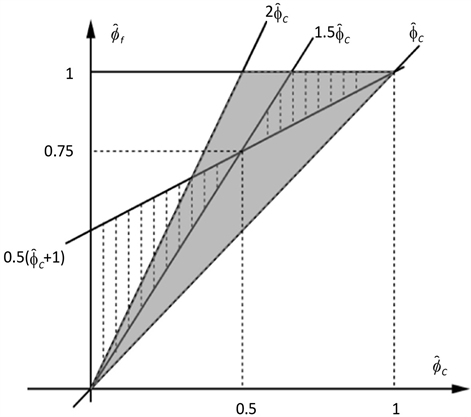Figure 2. The regions of the TVD (shaded) and BAIR (hatched)

2.3. Hermite插值构造新格式

$f\left(0\right)=0,f\left(\frac{1}{2}\right)=\frac{3}{4},f\left(1\right)=1$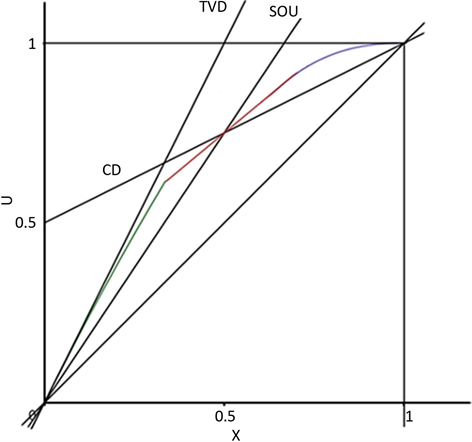Figure 3. The illustration of the NV line of the MCUI scheme in the BAIR region

${f}^{\text{Lax}}=\frac{1}{2}\left[f\left({\varphi }^{L}\right)+f\left({\varphi }^{R}\right)-\mathrm{max}|{f}^{\prime }\left(\varphi \right)|\left({\varphi }^{R}-{\varphi }^{L}\right)\right]$

$\frac{\partial {\stackrel{¯}{\phi }}_{j}}{\partial t}+\frac{1}{\text{Δ}x}\left({\stackrel{^}{f}}_{j+\frac{1}{2}}-{\stackrel{^}{f}}_{j-\frac{1}{2}}\right)=\frac{v}{\text{Δ}x}\left(\frac{{u}_{j+1}-2{u}_{j}+{u}_{j-1}}{\text{Δ}x}\right)$

2.4. 时间项的离散

$\frac{\text{d}\stackrel{¯}{\varphi }}{\text{d}t}=L\left(\varphi \right)$

$\left\{\begin{array}{l}{\varphi }^{\left(1\right)}={\varphi }^{n}+L\left({\varphi }^{n}\right)\\ {\varphi }^{\left(2\right)}=\frac{3}{4}{\varphi }^{n}+\frac{1}{4}\left[{\varphi }^{\left(1\right)}+L\left({\varphi }^{\left(1\right)}\right)\right]\\ {\varphi }^{n+1}=\frac{1}{3}{\varphi }^{n}+\frac{2}{3}\left[{\varphi }^{\left(2\right)}+L\left({\varphi }^{\left(2\right)}\right)\right]\end{array}$

3. 耦合Burgers方程组的数值算例

3.1. 算例1

$\frac{\partial u}{\partial t}-\delta \frac{{\partial }^{2}u}{\partial {x}^{2}}+\eta u\frac{\partial u}{\partial x}+\alpha \frac{\partial \left(uv\right)}{\partial x}=0,\text{\hspace{0.17em}}x\in \Omega =\left[a,b\right],\text{\hspace{0.17em}}t\in \left[0,T\right]$

$\frac{\partial v}{\partial t}-\mu \frac{{\partial }^{2}v}{\partial {x}^{2}}+\xi v\frac{\partial v}{\partial x}+\beta \frac{\partial \left(uv\right)}{\partial x}=0,\text{\hspace{0.17em}}x\in \Omega =\left[a,b\right],\text{\hspace{0.17em}}t\in \left[0,T\right]$

$x\in \left[-\pi ,\pi \right],\text{\hspace{0.17em}}\delta =\mu =1.0,\text{\hspace{0.17em}}\eta =\xi =-2.0,\text{\hspace{0.17em}}\alpha =\beta =1.0$

$u\left(x,t\right)=v\left(x,t\right)={\text{e}}^{-t}\mathrm{sin}\left(x\right)$

$u\left(0,t\right)=v\left(0,t\right)=\mathrm{sin}\left(x\right)$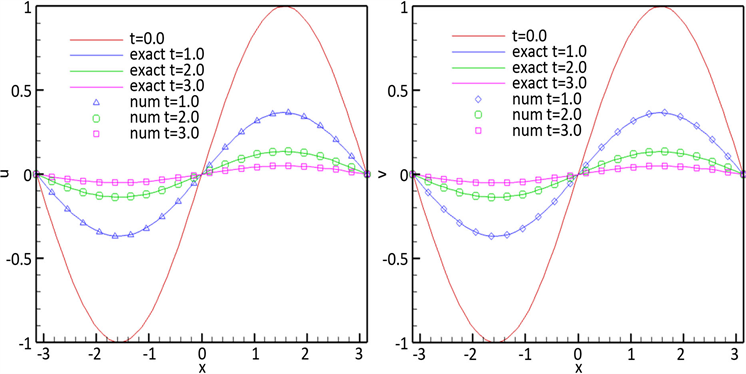Figure 4. The numerical solution and the exact solution of Example 1 at different time for α = β = 1.0

3.2. 算例2

$\frac{\partial u}{\partial t}-\delta \frac{{\partial }^{2}u}{\partial {x}^{2}}+\eta u\frac{\partial u}{\partial x}+\alpha \frac{\partial \left(uv\right)}{\partial x}=0,\text{\hspace{0.17em}}x\in \Omega =\left[a,b\right],\text{\hspace{0.17em}}t\in \left[0,T\right]$

$\frac{\partial v}{\partial t}-\mu \frac{{\partial }^{2}v}{\partial {x}^{2}}+\xi v\frac{\partial v}{\partial x}+\beta \frac{\partial \left(uv\right)}{\partial x}=0,\text{\hspace{0.17em}}x\in \Omega =\left[a,b\right],\text{\hspace{0.17em}}t\in \left[0,T\right]$

$u\left(x,t\right)={a}_{0}-2A\left(\frac{2\alpha -1}{4\alpha \beta -1}\right)\mathrm{tanh}\left(A\left(x-2At\right)\right)$

$v\left(x,t\right)={a}_{0}\left(\frac{2\beta -1}{2\alpha -1}\right)-2A\left(\frac{2\alpha -1}{4\alpha \beta -1}\right)\mathrm{tanh}\left(A\left(x-2At\right)\right)$

$A=\frac{4\alpha \beta -1}{4\alpha -2}$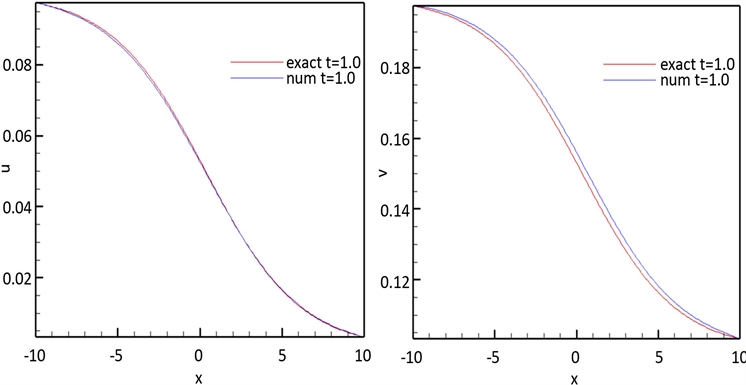Figure 5. The numerical solution and of Case 1 at t = 1.0 for α = 1.0, β = 2.0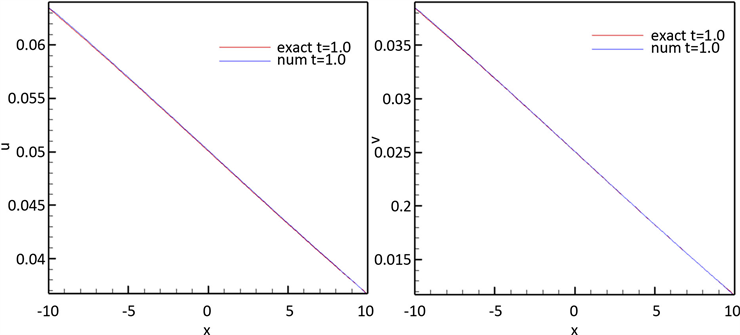Figure 6. The numerical solution and of Case 2 at t = 1.0 for α = 0.1, β = 0.3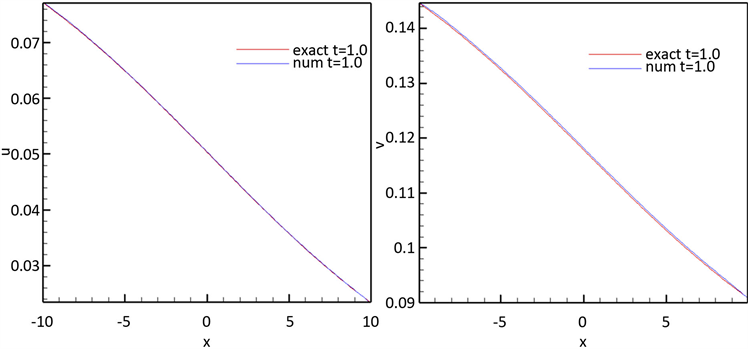Figure 7. The numerical solution and of Case 3 at t = 1.0 for α = 0.3, β = 0.03Table 2. Comparison of errors at different time for u(x,t) of Case 3Table 3. Comparison of errors at different time for v(x,t) of Case 3

4. 结论

  Esipov, S.E. (1995) Coupled Burgers’ Equations: A Model of Poly Dispersive Sedimentation. Physical Review E, 52, 3711-3718. https://doi.org/10.1103/PhysRevE.52.3711  Burgers, M. (1948) A Mathematical Model Illustrating the Theory of Turbulence. Advances in Applied Mechanics, 1, 171-199. https://doi.org/10.1016/S0065-2156(08)70100-5  Cole, D. (1951) On a Quasilinear Parabolic Equations Occurring in Aerodynamics. Quarterly of Applied Mathematics, 9, 225-236. https://doi.org/10.1090/qam/42889  Soliman, A. (2006) The Modiﬁed Extended Tanh-Function Method for Solving Burgers-Type Equations. Physica A: Statistical Mechanics and Its Applications, 361, 394-404. https://doi.org/10.1016/j.physa.2005.07.008  Abdou, M.A. and Soliman, A.A. (2005) Variational Iteration Method for Solving Burgers and Coupled Burgers Equations. Journal of Computational and Applied Mathematics, 181, 245-251. https://doi.org/10.1016/j.cam.2004.11.032  Deghan, M., Asgar, H. and Mohammad, S. (2009) The Solution of Linear and Nonlinear Systems of Volterra Functional Equations Using Adomian-Pade Technique. Chaos, Solitons & Fractals, 39, 2509-2521. https://doi.org/10.1016/j.chaos.2007.07.028  Mittal, R.C. and Arora, G. (2011) Numerical Solution of the Coupled Viscous Burgers’ Equation. Communications in Nonlinear Science and Numerical Simulation, 16, 1304-1313. https://doi.org/10.1016/j.cnsns.2010.06.028  Khater, A.H., Temsah, R.S. and Hassan, M.M. (2008) A Chebyshev Spectral Collocation Method for Solving Burgers-Type Equations. Journal of Computational and Applied Mathematics, 222, 333-350. https://doi.org/10.1016/j.cam.2007.11.007  Rashid, A. and Ismail, A. (2009) A Fourier Pseudo Spectral Method for Solving Coupled Viscous Burgers’ Equations. Computational Methods in Applied Mathematics, 9, 412-420. https://doi.org/10.2478/cmam-2009-0026  Mittal, R.C. and Jiwari, R. (2012) Differential Quadrature Method for Numerical Solution of Coupled Viscous Burger’s Equation. International Journal for Computational Methods in Engineering Science and Mechanics, 13, 88-92. https://doi.org/10.1080/15502287.2011.654175  Mohanty, R.K., Dai, W. and Han, F. (2015) Compact Operator Method of Accuracy Two in Time and Four in Space for the Numerical Solution of Coupled Viscous Burgers’ Equations. Applied Mathematics and Computation, 256, 381-393. https://doi.org/10.1016/j.amc.2015.01.051  Arminjon, P. and Beauchamp, C. (1979) Numerical Solution of Burgers’ Equations in Two-Space Dimensions. Computer Methods in Applied Mechanics and Engineering, 19, 351-365. https://doi.org/10.1016/0045-7825(79)90064-1  Wei, G.W., Zhang, D.S., Kouri, D.J. and Hoffman, D.K. (1998) Distributed Approximation Functional Approach to Burgers’ Equation in One and Two Space Dimensions. Computer Physics Communications, 111, 93-109. https://doi.org/10.1016/S0010-4655(98)00041-1  Srivastava, V.K., Awasthi, M.K. and Tamsir, M. (2013) A Fully Implicit Finite-Difference Solution to One Dimensional Coupled Nonlinear Burgers’ Equations. International Journal of Mathematical, Computational Sciences and Engineering, 7, 283-287. https://doi.org/10.1142/S1793557113500587  Rashida, A., Abbas, M., Izani, A. and Ismail, A.A.M. (2014) Numerical Solution of the Coupled Viscous Burgers Equations by Chebyshev-Legendre Pseudo-Spectral Method. Applied Mathematics and Computation, 245, 372-381. https://doi.org/10.1016/j.amc.2014.07.067  Kumar, M. and Pandit, S. (2014) A Composite Numerical Scheme for the Numerical Simulation of Coupled Burgers’ Equation. Computer Physics Communications, 185, 809-817. https://doi.org/10.1016/j.cpc.2013.11.012  Bhatt, H.P. and Khaliq, A.Q.M. (2016) Fourth-Order Compact Schemes for the Numerical Simulation of Coupled Burgers’ Equation. Computer Physics Communications, 200, 117-138. https://doi.org/10.1016/j.cpc.2015.11.007  Leonard, B.P. (1988) Simple High-Accuracy Resolution Program for Convective Modeling of Discontinuities. International Journal for Numerical Methods in Fluids, 8, 1291-1318. https://doi.org/10.1002/fld.1650081013  Wei, J.J., Yu, B. and Tao, W.Q. (2003) A New High-Order-Accurate and Bounded Scheme for Incompressible Flow. Numerical Heat Transfer, Part B: Fundamentals, 24, 353-337.  Hou, P.L. (2003) Refinement of the Convective Boundedness Criterion of Gaskell and Lau. Engineering Computations: International Journal for Computer-Aided Engineering, 20, 1023-1043. https://doi.org/10.1108/02644400310503008